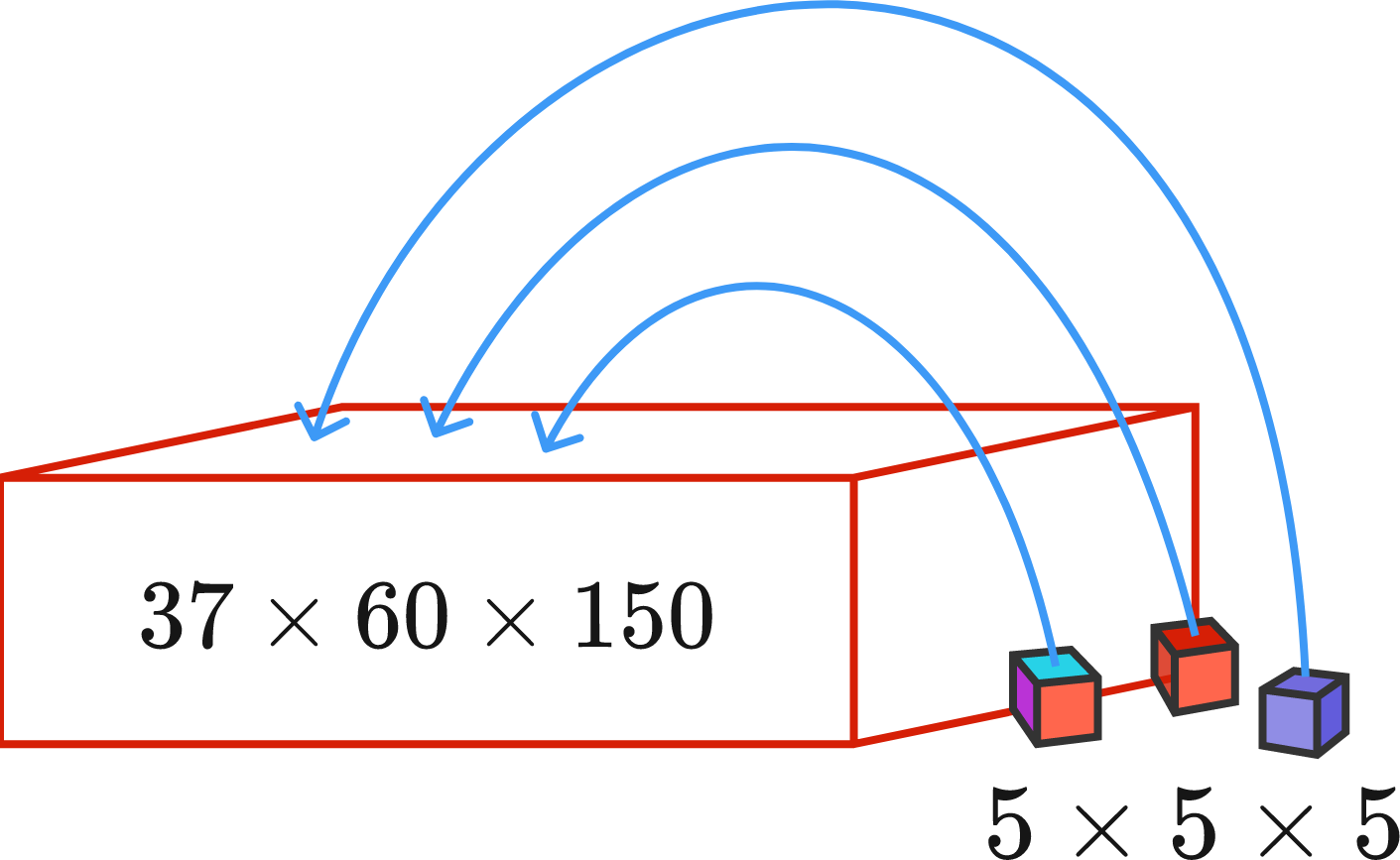# Filling in Cuboid with Cubes

Geometry Level 1

Suppose you want to fill a $150\times60\times37$ cuboid with $5 \times 5 \times 5$ cubes. To calculate how many fit, you divide the two volumes:

$\dfrac{37\times60\times150}{5\times5\times5} = 2664.$

You conclude that given optimal placement, 2664 of the cubes fit inside the cuboid. Is this correct?

Assume that all the faces of the cubes are in parallel with the faces of the cuboid.×